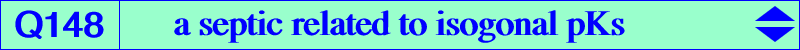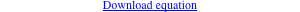too complicated to be written here. Click on the link to download a text file.X(1), X(2), X(3), X(1113), X(1114) excenters vertices of the CircumTangential triangle vertices of the cevian triangle of X(3)Let (K) be the cubic pK(X6, P) with pivot P, isopivot P* and tertiary pivot the P-Ceva conjugate P/P* of P*. The polar conic of P is the diagonal rectangular hyperbola (D) passing through P and the in/excenters of ABC. The polar conic of P* is the circum-conic (C) passing through P and P*. It is a rectangular hyperbola if and only if P and then P* lie on the McCay cubic K003. The polar conic of P/P* is the bicevian conic (B) passing through P*, P/P* and the vertices of the cevian triangle of P. It is a rectangular hyperbola if and only if P lies on the septic Q148. A, B, C are triple points on Q148. The tangents at A are the two bisectors and the line passing through the trace of the orthic axis on BC. Q148 meets the Euler line at X(2), X(3), X(1113), X(1114) and three other points E1, E2, E3 that lie on the cubics (K1) = pK(X4, X107) and (K2) = pK(X648, X264). The three polar conics of P, P*, P/P* are all proper rectangular hyperbolas if and only if P = X(3) hence (K) = K003, (D) = Stammler hyperbola, (C) = Jerabek hyperbola, (B) = C(X3, X15352) passing through X(4), X(417), X(1075).